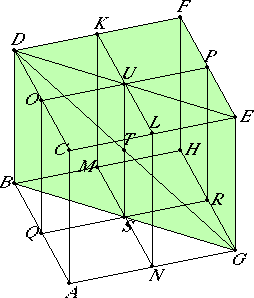# Proposition 38

If the sides of the opposite planes of a cube are bisected, and the planes are carried through the points of section, then the intersection of the planes and the diameter of the cube bisect one another.

Let the sides of the opposite planes CF and AH of the cube AF be bisected at the points K, L, M, N, O, Q, P, and R, and through the points of section let the planes KN and OR be carried. Let US be the common section of the planes, and DG the diameter of the cube AF.

I say that UT equals TS, and DT equals TG.

Join DU, UE, BS, and SG.I.29

Then, since DO is parallel to PE, therefore the alternate angles DOU and UPE equal one another.

I.4

Since DO equals PE, and OU equals UP, and they contain equal angles, therefore the base DU equals the base UE, the triangle DOU equals the triangle PUE, and the remaining angles equal the remaining angles. Therefore the angle OUD equals the angle PUE.

I.14

Therefore DUE is a straight line. For the same reason BSG is also a straight line, and BS equals SG.

XI.9

Now, since CA equals and is parallel to DB, while CA also equals and is parallel to EG, therefore DB equals and is parallel to EG.

I.33

And the straight lines DE and BG join their ends, therefore DE is parallel to BG.

Therefore the angle EDT equals the angle BGT, for they are alternate, and the angle DTU equals the angle GTS.

I.26

Therefore DTU and GTS are two triangles which have two angles equal to two angles and one side equal to one side, namely that opposite one of the equal angles, that is, DU equals GS, for they are the halves of DE and BG, therefore the remaining sides equal the remaining sides. Therefore DT equals TG, and UT equals TS.

Therefore, if the sides of the opposite planes of a cube are bisected, and the planes are carried through the points of section, then the intersection of the planes and the diameter of the cube bisect one another.

Q.E.D.

## Guide

This proposition takes care of a specific situation that occurs in proposition XIII.17. In XIII.17 a dodecahedron is constructed based on a cube, and the fact proven here in XI.38 is needed to show that the point T where SU intersects DG is the center of a sphere circumscribing the cube.

There are a couple of details missing from this proof. For instance, it is not shown that KL and MN actually lie in one plane, and that the line SU actually intersects the line DG.